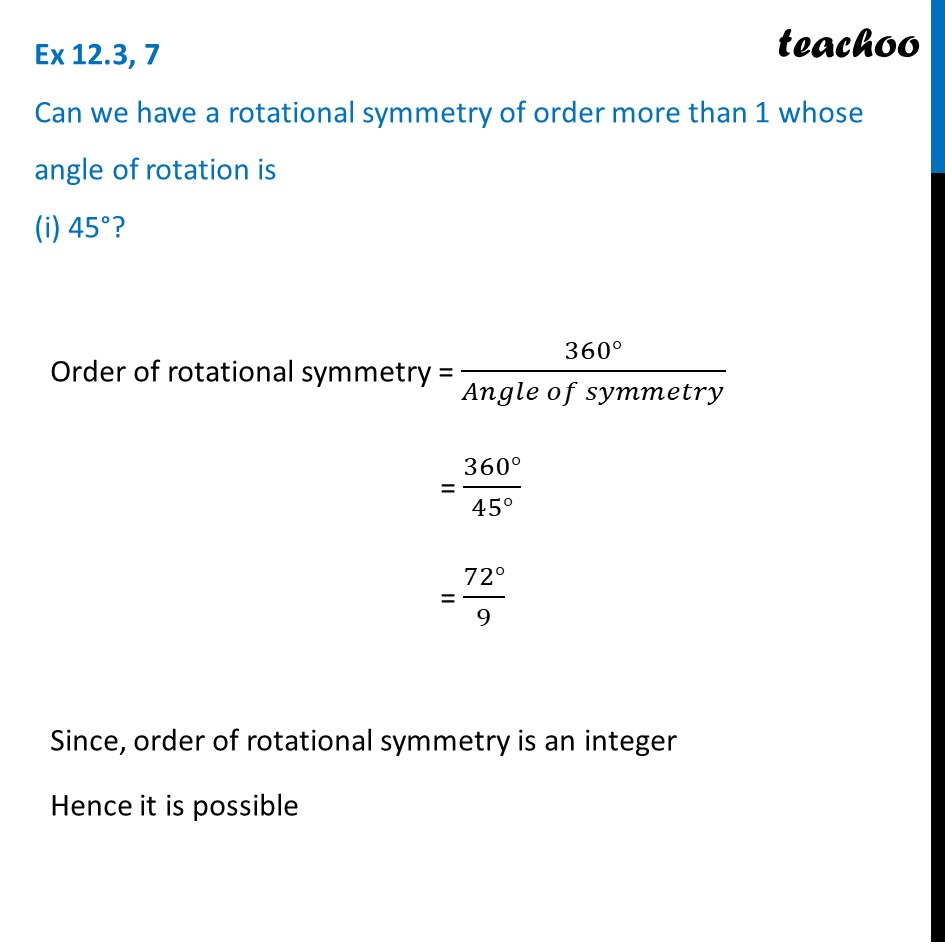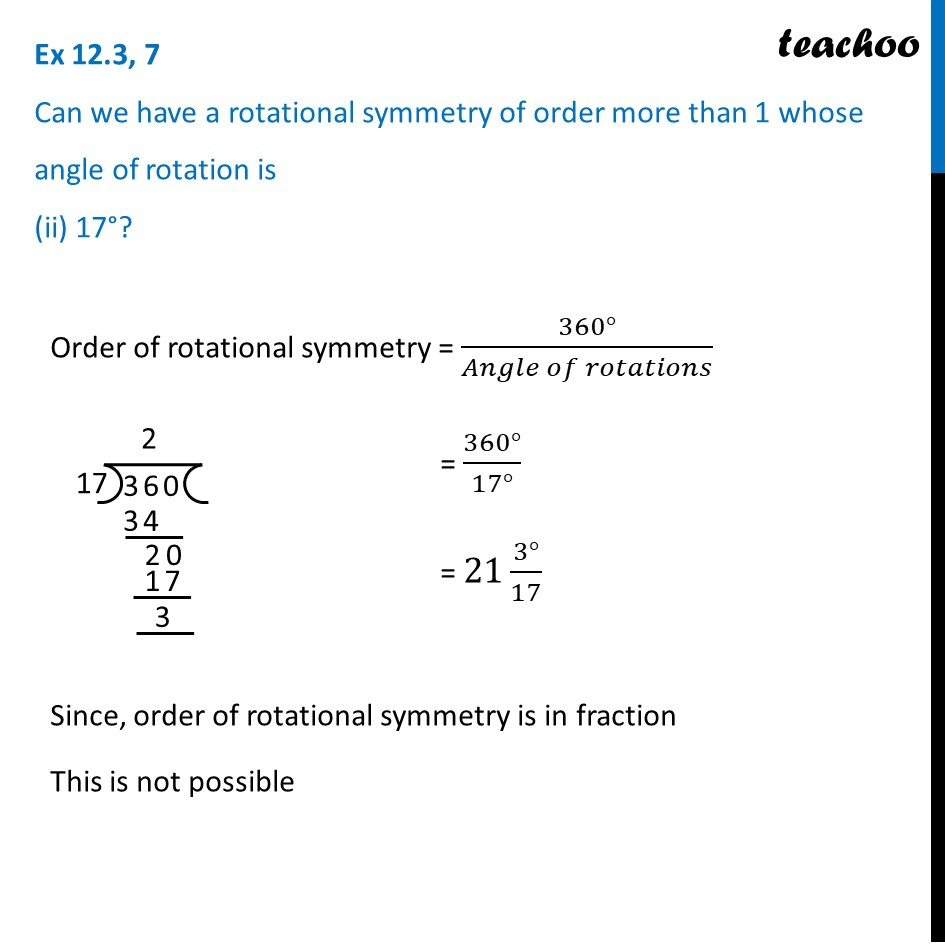Ex 12.3

Chapter 12 Class 7 Symmetry
Serial order wiseLearn in your speed, with individual attention - Teachoo Maths 1-on-1 Class

### Transcript

Ex 12.3, 7 Can we have a rotational symmetry of order more than 1 whose angle of rotation is (i) 45°? Order of rotational symmetry = (360°)/(𝐴𝑛𝑔𝑙𝑒 𝑜𝑓 𝑠𝑦𝑚𝑚𝑒𝑡𝑟𝑦) = (360°)/(45°) = (72°)/9 Since, order of rotational symmetry is an integer Hence it is possible Ex 12.3, 7 Can we have a rotational symmetry of order more than 1 whose angle of rotation is (ii) 17°?Order of rotational symmetry = (360°)/(𝐴𝑛𝑔𝑙𝑒 𝑜𝑓 𝑟𝑜𝑡𝑎𝑡𝑖𝑜𝑛𝑠) = (360°)/(17°) = 21 (3°)/17 Since, order of rotational symmetry is in fraction This is not possible